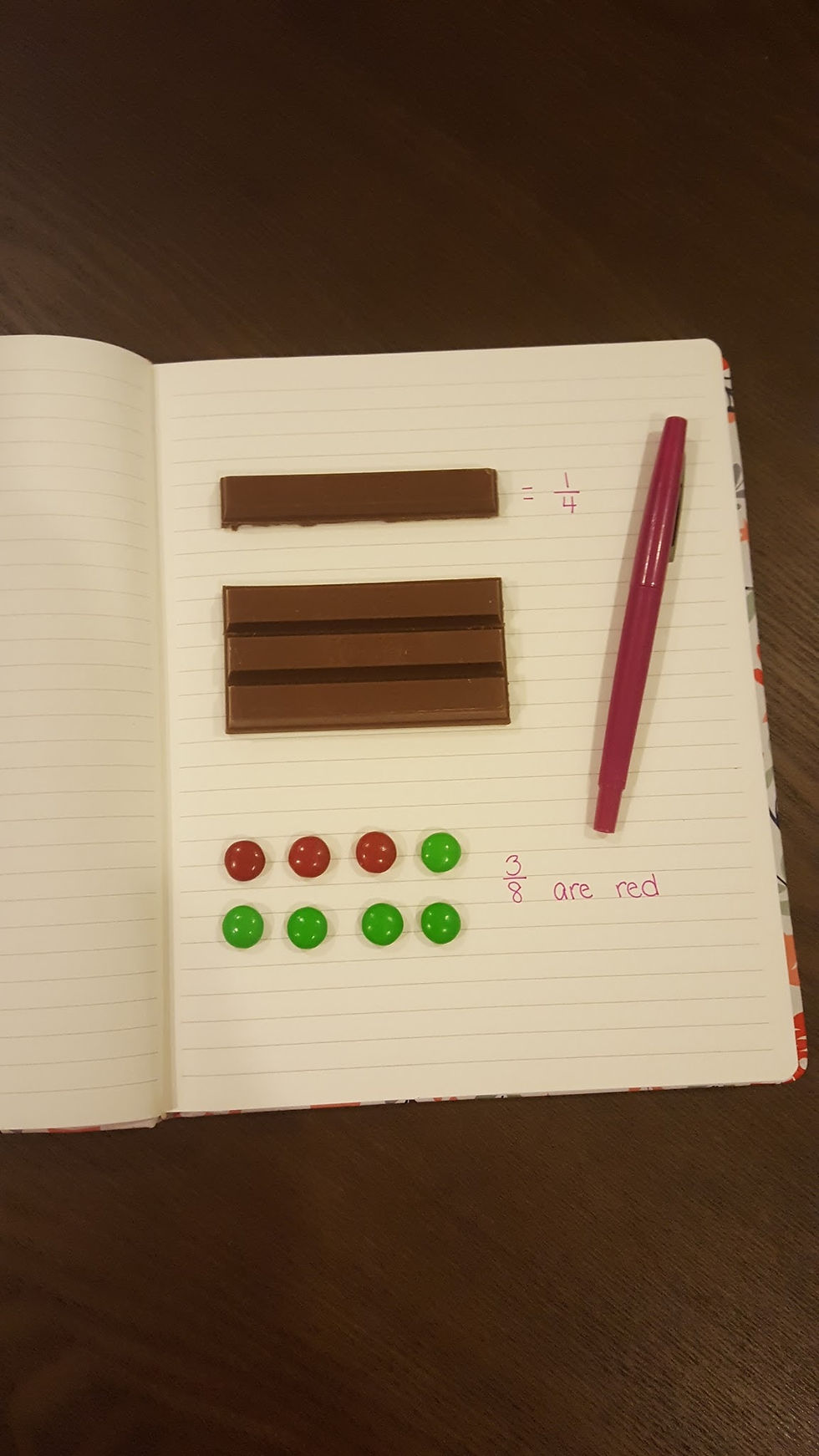top of pageThere are so many incredible ways to teach fractions. Using manipulatives, visual models, number lines, and then of course the favorite in my classroom is using food! Fractions are challenging for many students to grasp so I find the more real-life I can make it, the better student learning will be. My experience with fraction instruction has been as simple as identifying fractions with my third graders to multiplying and dividing fractions with my fifth graders (read my blog about Fifth Graders and Fractions). Yet with both age groups I found that I kept referencing food, recipes, and items that might come in sets (i.e. crayons, blocks, balloons, etc.) to help students make connections to examples of when fractions might be present in everyday life experiences.

In third grade, I always introduce fractions with food! The first concept students must learn and understand is that a fraction is a part of a whole There are some great foods that you can use that already have fractional parts: Kit-Kats, Hershey Bars, oranges, Graham Crackers, pizza, etc. I usually take a Kit-Kat bar, break it into parts and show the students visually the whole Kit-Kat and what happens if I eat one part or two parts of the bar. We discuss the fraction of the whole that I would be eating. We continue this learning with other foods and examples. Eating slices of pizza is always one that all the students can relate to or the fraction of M&M’s that are a certain color in a set! The first few days of fractions is my time to use food and manipulatives galore, in order to build a really strong foundation of the concept of fractions as equal parts of a whole. Then we begin using visual models and set diagrams to continue practicing the concept.

The learning progression continues in third grade as students begin learning more about number lines and also equivalent fractions. I always find that number lines and equivalent fractions are the most difficult concepts for students. Students usually have difficulty naming fractions on a number line and creating their own number line model. Equivalent fractions are more abstract for students and it can be difficult for them to see the connection with how it is relevant in the real world. For both of these concepts, I have created many visual resources to reinforce student learning. Read my blog posts Ugh! Fractions on a Number Line and Equivalent Fractions for more resources and ideas for teaching fractions and check out the Fifth Grade Fractions blog for activities and resources for the older kids!

Fractions are an abstract concept. Try to give your students as many concrete or pictorial representations of fractions as possible. It is important for students to have a strong understanding of what a fraction is in order to understand equivalent fractions or operations involving fractions.

Tags: